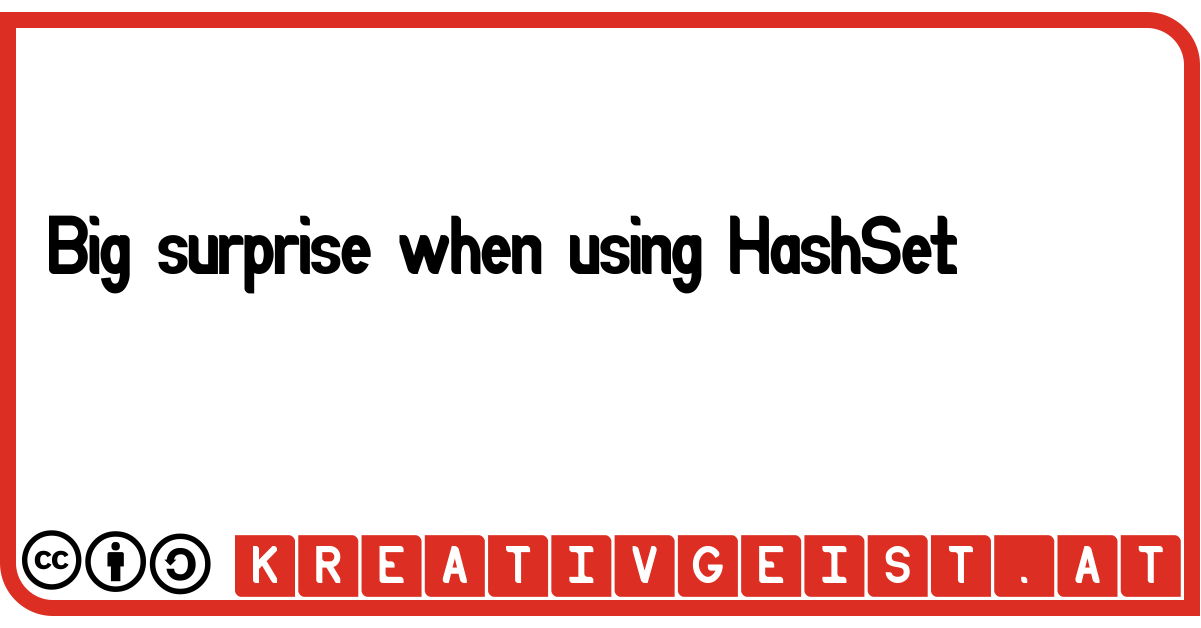# Confusing HashSets

What output do you expect? And why?

## Introduction

Sometime in a developer's life things don't work as expected. This example is not real-life, I discovered a few notices about how hashMap and hashSet works while reading the documentation of Java.
As a good practice it is always mentioned to implement equals() in data-objects. As a side-effect you are gently forced to also implement hashCode(). In common IDEs it is usual to use the same fields to calculate `hashCode()` as used in `equals()`. But is this reasonable and prudent?

## Define a sample class

``````
public class Item {
public int value;

@Override
public boolean equals(Object o) {
boolean result = false;
if (o instanceof Item) {
Item that = (Item) o;
result = (this.value == that.value);
}
return result;
}

@Override
public int hashCode() {
int hash = 7;
hash = 97 * hash + this.value;
return hash;
}
}
``````
The class `Item` only consists of a single attribute called `value`. The `equals()`-function compares the type and if the value is equal. The `hashCode()`-function also uses the same attribute to calculate its value.

## Putting an item into a HashSet

Now let's define a HashSet and put an item into it.
``````
HashSet <Item> set = new HashSet<>();
Item item = new Item();
item.value = 1;

System.out.println(set.contains(item));

``````
Also as expected, the set contains the element. So we change the value of the item.
``````
item.value++;
System.out.println(set.contains(item));
``````
But what happend now? The item is not inside the HashSet! Even the element has the same reference as the inserted element. If you iterate over the set and compare its contained item with `item`, they are equal.
So what happened?

## Look into the documentation of Java

If we have a look at the Java documentation of the HashSet-function contains() it says:
"Returns true if this set contains the specified element. More formally, returns true if and only if this set contains an element e such that Objects.equals(o, e)."
If we add the item again to the set via `set.add(item);`, the item should not be added as also claimed in the documentation of HashSet's add-function.
"Adds the specified element to this set if it is not already present. More formally, adds the specified element e to this set if this set contains no element e2 such that Objects.equals(e, e2). If this set already contains the element, the call leaves the set unchanged and returns false."

## The cause

The cause of this problem is found in the documentation of hashCode. There it says:
"Whenever it is invoked on the same object more than once during an execution of a Java application, the hashCode method must consistently return the same integer, provided no information used in equals comparisons on the object is modified. This integer need not remain consistent from one execution of an application to another execution of the same application."
In this example we did not obey that rule. We changed the hashCode by changing `value`, because it is recalculated every time we call it.

## The solution

Since the equals-function says that two equal items must also return the same hashCode, we cannot calculate a hashCode when the object is created. That would violate the rule.
The only chance we have to fix it, is to keep the object immutable or at least the attributes that were used to calculate the hashCode. Hence, the only candidates for attributes to calculate the hashCode are marked with `final`.
In the worst case there is no invariant attribute, then the hashCode is a constant. In that case the data-structure HashSet is very slow in finding elements. In that case an implementation for the `Set` should be used that not depends on a hashCode.

## Conclusion

HashSets can cause extraordinary results, when not using them with immutable elements. Therefore it is highly recommended to implement the hashCode-function only with attributes that were marked final.